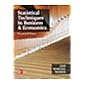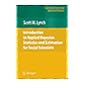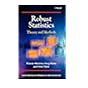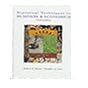Normal view

# Introduction to applied Bayesian statistics and estimation for social scientists / Scott M. Lynch.

Material type:TextLanguage: English Publisher: New York : Springer, c2007Description: xxviii, 357 p. : ill. ; 24 cmISBN: 9780387712642 (hardcover : alk. paper)DDC classification: 519.5 LYI LOC classification: HA29 | .L973 2007Online resources: Table of contents | OCLC | E-book Fulltext
Contents:
1. Introduction -- 2. Probability theory and classical statistics -- 3. Basics of Bayesian statistics -- 4. Modern model estimation part 1 : Gibbs sampling -- 5. Modern model estimation part 2 : Metropolis-Hastings sampling -- 6. Evaluating Markov chain Monte Carlo algorithms and model fit -- 7. The linear regression model -- 8. Generalized linear models -- 9. Introduction to hierarchical models -- 10. Introduction to multivariate regression models -- 11. Conclusion -- A. Background mathematics -- B. The central limit theorem, confidence intervals, and hypothesis tests.
Summary: Lynch covers the complete process of Bayesian statistical analysis in great detail from the development of a model through the process of making statistical inference. The key feature of the book is that it covers models that are most commonly used on social science research.
Tags from this library: No tags from this library for this title.Average rating: 0.0 (0 votes)
Item type Current location Collection Call number Copy number Status Date due Barcode Item holdsE-Book
E-book
Non-fiction 519.5 LYI 2007 (Browse shelf) Not For LoanText
Reserve Section
Non-fiction 519.5 LYI 2007 (Browse shelf) C-1 Not For Loan 25614Text
Circulation Section
Non-fiction 519.5 LYI 2007 (Browse shelf) C-2 Available 26049
Total holds: 0
##### Browsing EWU Library shelves, Shelving location: E-book Close shelf browser519.5 LIS 2012 Statistical techniques in business & economics / 519.5 LIS 2012 Statistical techniques in business & economics / 519.5 LIS 2018 Statistical techniques in business & economics / 519.5 LYI 2007 Introduction to applied Bayesian statistics and estimation for social scientists / 519.5 MAR 2006 Robust statistics : 519.5 MAS 1996 Statistical techniques in business and economics / 519.5 MAT 2008 Mathematical statistics with applications /

Includes bibliographical references (p. -351) and index.

1. Introduction --
2. Probability theory and classical statistics --
3. Basics of Bayesian statistics --
4. Modern model estimation part 1 : Gibbs sampling --
5. Modern model estimation part 2 : Metropolis-Hastings sampling --
6. Evaluating Markov chain Monte Carlo algorithms and model fit --
7. The linear regression model --
8. Generalized linear models --
9. Introduction to hierarchical models --
10. Introduction to multivariate regression models --
11. Conclusion --
A. Background mathematics --
B. The central limit theorem, confidence intervals, and hypothesis tests.

Lynch covers the complete process of Bayesian statistical analysis in great detail from the development of a model through the process of making statistical inference. The key feature of the book is that it covers models that are most commonly used on social science research.

Applied Statistics

There are no comments for this item.